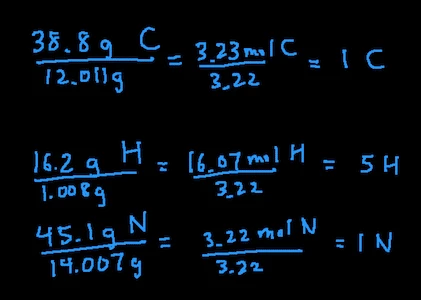## How to Find Empirical and Molecular Formula Given Mass Percent

In this post, you’ll learn how to find the empirical and molecular formula of an unknown compound when given the mass percent of the compound.

First, we’ll start by going over all the steps. Then we’ll apply the step by going through an example.

### Steps for Finding The Empirical Formula Given Mass Percent

1. Change % of each element into grams (for example, if the compound contains 40% carbon, then change it to 40 g carbon)
2. Convert grams of each element into moles by dividing grams by molar mass
3. Divide all moles by the smallest number of moles
4. If the moles are all whole numbers, then you’re done and that’s your empirical formula
5. If not, multiply all moles by number (usually 2 or 3) such that everything is a whole number

### Example

Lets work through this example together and go through it step by step.

What is the empirical formula for a compound containing 38.8% carbon, 16.2 % hydrogen, and 45.1% nitrogen?

Steps

1. Change % in to grams: 38.8 g carbon, 16.2 g hydrogen, and 45.1 g nitrogen
2. Convert grams into moles: 38.8 g C / 12.011 = 3.23 mol C, 16.2 g H / 1.008 = 16.07 mol H, 45.1 g N / 14.007 = 3.22 mol N
3. Divide all moles by smallest moles: in this case, the smallest moles is 3.22 mol of N so we’ll divide all the moles by 3.22. This leaves us with 1 C, 5 H, 1 N
4. Since the moles of all the elements are whole numbers, we are done. That means the empirical formula of this compound is CH5N### Steps for Finding The Molecular Formula from Empirical Formula

To be able to find the molecular formula, you’ll need to given the molar mass of the compound.

1. Divide the molar mass of the compound by the molar mass of the empirical formula. This should give you a whole number
2. Multiply all the subscripts of the empirical formula by the whole number. This will give you the molecular formula.

### Example

Let’s continue with the example we were working through. If the molar mass of the unknown compound is 62.11 g/mol, what is the molecular formula.

Steps

1. First determine the molar mass of the empirical formula, which is 31.0571 g/mol. Divide 62.11 by 31.0571 and this will give you 2.
2. Next, multiply all the subscripts in CH5N by 2. This gives us C2H10N2.

And that’s it! Follow the steps, practice, and you’ll be able to solve these types of problems with no problem. Soon you’ll be conquering chemistry.